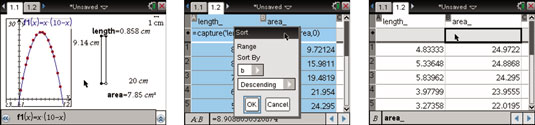##### TI-Nspire For DummiesAfter you have both graphical and numerical results available on the TI-Nspire, you can analyze them to discover find and graph an equation to model a scatter plot or to sort the data to find the length that produces a maximum area, among other things.

• Find and graph an equation to model the scatter plot. An inspection of the scatter plot suggests that a quadratic equation can be used to model the data. In fact, if you let x represent the length of the parabola, you can find an expression for the area in terms of x, knowing that the perimeter has a fixed value.

In the example, there is a fixed perimeter of 20 centimeters. Therefore, the width of the rectangle must be 10 – x. The formula for the area is the product of length and width, or area(x) = x(10 – x). Type this equation into f1(x) on the entry line to verify that it goes through the scatter plot. Don’t forget to include a multiplication sign between the length and the width! See the first screen.

• Sort the data to find the length that produces a maximum area. For those of you who are more into the numbers, move to the Lists & Spreadsheet application, highlight columns A and B, and perform a descending sort by column B. The second screen shows how to configure the Sort dialog box. The third screen shows that, for my example, the area is maximized for a length of approximately 4.833 centimeters.Formulas contained in the formula row are deleted anytime you do a sort on that column. Press [CTRL][ESC] to undo the sort and restore the formulas.